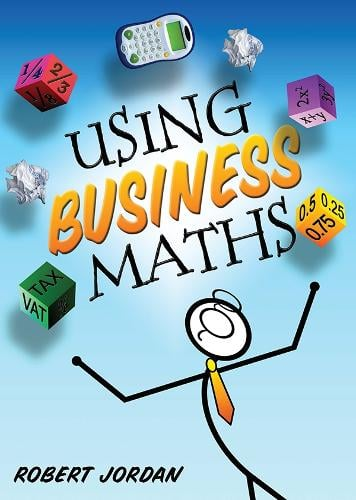•(author)
£15.99
Paperback 240 Pages / Published: 17/05/2013
• We can order this

Usually dispatched within 2 weeks

New student-friendly textbook introducing common calculations encountered regularly in both personal and business scenarios.

Presents calculations and problems in a clear and logical manner using humour and illustrations to aid understanding.
Positions the concepts of maths and calculations in the real world and demonstrates the importance of maths in everyday life.
Demonstrates how to use a calculator accurately to perform extended calculations, as well as how to perform simple calculations without a calculator.
Clearly explains percentages, law of indices, rules of logarithms, simple and compound interest, payroll and associated taxes, VAT, net profit and loss and algebra, including quadratic equations.
Illustrates how to mathematise scenarios, make an initial model, decide on appropriate mathematical techniques and tools, examine relationships and assumptions, make adjustments and present results and conclusions.

Specifically written for the revised NFQ Level 4 modules Business Calculations (4N2279) and Functional Mathematics (4N2138) modules, as awarded by QQI.

Publisher: Gill
ISBN: 9780717157150
Number of pages: 240
Weight: 366 g
Dimensions: 245 x 172 x 10 mm